RandomWorksheets.com

A simple tool to create free randomized printable worksheets for kids.

Pre-K Worksheets

Single Number Counting Objects Worksheet
Number Counting Worksheet - With Right and Wrong Answers
Number Counting Worksheet - Without Answers
Number Counting Worksheet - With Right and Wrong Answers (15-40)
Number Counting Worksheet - Without Answers (15-40)
Alphabet Letter Tracing Worksheet (A-Z)
Single Letter Tracing Worksheet
Mixed Case Alphabet Letter Tracing Worksheet
Number Tracing Worksheet (1-10, and 1-20)

Elementary Worksheets

Arithmetic Problems Worksheet (+ - x)
Commmon Core Addition Worksheet
Commmon Core Comparison Worksheet
Commmon Core Comparison Worksheet (Alternative Format 1)
Commmon Core Comparison Worksheet (Alternative Format 2)

Acknowledgements

Single Number Counting Objects Worksheet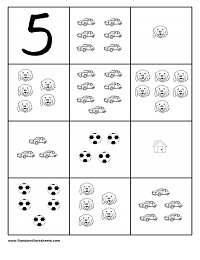"Circle which of these boxes contain __ number of things." This worksheet presents a single number (learning objective) followed by a series of 11 images with various numbers of figures allowing the student to circle the set(s) which contain the learning objective number of figures.

Number:
Occurrences:
Total Pages:

Instructions

Number: the learning objective (i.e. the number being taught). This is a number between 1 and 10. The default number selected is 5.

Occurrences: the number of times to show the learning objective number. For example, if Occurrences is set to 4, and the learning objective Number is set to 5, then 4 of the 11 images displayed will contain 5 figures (i.e. correct choices for the students to circle); the other 7 images will contain a different number of figures (i.e. incorrect choices). The minimum value for this is 0 (i.e. none of the images shown will contain the learning objective Number of figures); the maximum value for this is 11 (i.e. all images displayed will contiain the learning objective Number of figures). The default value selected is 4 (i.e. 4 of the 11 images shown will contain the learning objective Number of figures).

Total Pages: the total number of pages to generate. If printing double-sided (duplex), set this to an even number so that the last page prints on both sides.

Generate PDF: use this button to generate a printable PDF file of the worksheet pages with the desired learning objectives.

Show as Web Page: use this button if you do not have a PDF viewer and would like to display the worksheet pages as a single web page. You will need to manually adjust page margins when printing using this option.

Number Counting Worksheet - With Right and Wrong Answers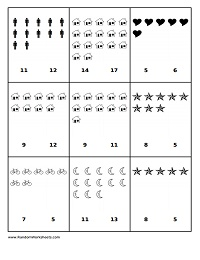This worksheet presents a series of figures with two numbers below it (a right answer, and a wrong answer).

Lower Limit:
Upper Limit:
Tolerance:
Total Pages:

Instructions

Lower Limit: the lower limit of the learning objective (i.e. the lowest number to show). This is typically set to 1, 5, 10, or 15. This number must be less than the Upper Limit.

Upper Limit: the upper limit of the learning objective (i.e. the highest number to show). This is typically set to 10, 15, or 20. This number must be greater than the Lower Limit.

Tolerance: the maximum difference between the correct and incorrect answer in each box (i.e. if this is set to 3, and the right answer to a problem is 5, then the range of the wrong answer presented will be between 2 and 8.). This is typically set to 3 or 5 in order to avoid an obvious wrong answer.

Total Pages: the total number of pages to generate. If printing double-sided (duplex), set this to an even number so that the last page prints on both sides.

Generate PDF: use this button to generate a printable PDF file of the worksheet pages with the desired learning objectives.

Show as Web Page: use this button if you do not have a PDF viewer and would like to display the worksheet pages as a single web page. You will need to manually adjust page margins when printing using this option.

Number Counting Worksheet - Without Answers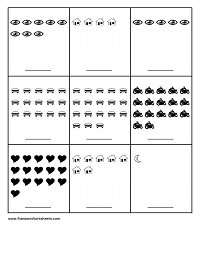This worksheet presents a series of figures with a blank space in which to write the correct answer.

Lower Limit:
Upper Limit:
Total Pages:

Instructions

Lower Limit: the lower limit of the learning objective (i.e. the lowest number to show). This is typically set to 1, 5, 10, or 15. This number must be less than the Upper Limit.

Upper Limit: the upper limit of the learning objective (i.e. the highest number to show). This is typically set to 10, 15, or 20. This number must be greater than the Lower Limit.

Total Pages: the total number of pages to generate. If printing double-sided (duplex), set this to an even number so that the last page prints on both sides.

Generate PDF: use this button to generate a printable PDF file of the worksheet pages with the desired learning objectives.

Show as Web Page: use this button if you do not have a PDF viewer and would like to display the worksheet pages as a single web page. You will need to manually adjust page margins when printing using this option.

Number Counting Worksheet - With Right and Wrong Answers (15 to 40)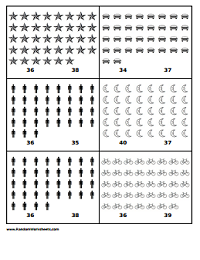Same as above -- expect with larger numbers (displays 6 per page instead of 9 per page) -- this worksheet presents a series of figures with two numbers below it (a right answer, and a wrong answer).

Lower Limit:
Upper Limit:
Tolerance:
Total Pages:

Instructions

Lower Limit: the lower limit of the learning objective (i.e. the lowest number to show). This is typically set to 15, 20, 25, 30, or 35. This number must be less than the Upper Limit.

Upper Limit: the upper limit of the learning objective (i.e. the highest number to show). This is typically set to 20, 25, 30, 35, or 40. This number must be greater than the Lower Limit.

Tolerance: the maximum difference between the correct and incorrect answer in each box (i.e. if this is set to 3, and the right answer to a problem is 25, then the range of the wrong answer presented will be between 22 and 28.). This is typically set to 3 or 5 in order to avoid an obvious wrong answer.

Total Pages: the total number of pages to generate. If printing double-sided (duplex), set this to an even number so that the last page prints on both sides.

Generate PDF: use this button to generate a printable PDF file of the worksheet pages with the desired learning objectives.

Show as Web Page: use this button if you do not have a PDF viewer and would like to display the worksheet pages as a single web page. You will need to manually adjust page margins when printing using this option.

Number Counting Worksheet - Without Answers (15 to 40)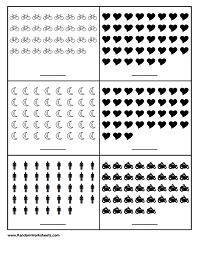Same as above -- expect with larger numbers (displays 6 per page instead of 9 per page) -- this worksheet presents a series of figures with a blank space in which to write the correct answer.

Lower Limit:
Upper Limit:
Total Pages:

Instructions

Lower Limit: the lower limit of the learning objective (i.e. the lowest number to show). This is typically set to 15, 20, 25, 30, or 35. This number must be less than the Upper Limit.

Upper Limit: the upper limit of the learning objective (i.e. the highest number to show). This is typically set to 20, 25, 30, 35 or 40. This number must be greater than the Lower Limit.

Total Pages: the total number of pages to generate. If printing double-sided (duplex), set this to an even number so that the last page prints on both sides.

Generate PDF: use this button to generate a printable PDF file of the worksheet pages with the desired learning objectives.

Show as Web Page: use this button if you do not have a PDF viewer and would like to display the worksheet pages as a single web page. You will need to manually adjust page margins when printing using this option.

Alphabet Letter Tracing Worksheet (A-Z)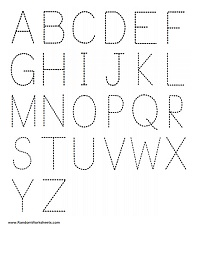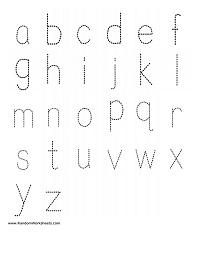This worksheet prints the alphabet using large dotted letters for tracing.

Case:
UPPER CASE
lower case
Total Pages:

Instructions

Case: Select whether you want the alphabet printed using UPPER CASE or lower case letters.

Total Pages: the total number of pages to generate. If printing double-sided (duplex), set this to an even number so that the last page prints on both sides.

Generate PDF: use this button to generate a printable PDF file of the worksheet pages with the desired learning objectives.

Show as Web Page: use this button if you do not have a PDF viewer and would like to display the worksheet pages as a single web page. You will need to manually adjust page margins when printing using this option.

Single Letter Tracing Worksheet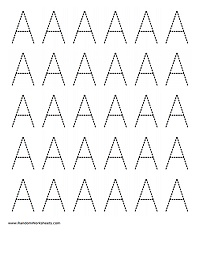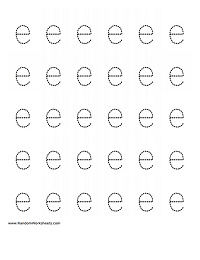This worksheet prints a single letter of the alphabet using large dotted letters for tracing.

Letter:
Case:
UPPER CASE
lower case
Total Pages:

Instructions

Letter: Select the letter to print. *** NOTE: If you select "A-Z (26 pages)" then all 26 letters of the alphabet will be printed (1 per page).

Case: Select whether you want the alphabet printed using UPPER CASE or lower case letters.

Total Pages: the total number of pages to generate. If printing double-sided (duplex), set this to an even number so that the last page prints on both sides. *** NOTE: If you selected "A-Z (26 pages)" for the Letter, then this selection for Total Pages will be ignored becasue all 26 letters of the alphabet will be printed (1 per page).

Generate PDF: use this button to generate a printable PDF file of the worksheet pages with the desired learning objectives.

Show as Web Page: use this button if you do not have a PDF viewer and would like to display the worksheet pages as a single web page. You will need to manually adjust page margins when printing using this option.

Mixed Case Alphabet Letter Tracing Worksheet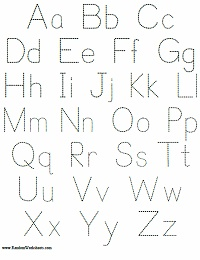Number Tracing Worksheet (1-10, and 1-20)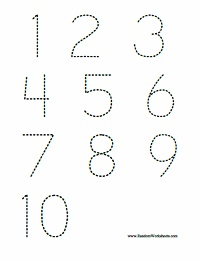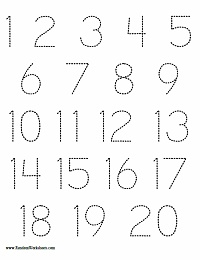Arithmetic Problems Worksheet (+ - x)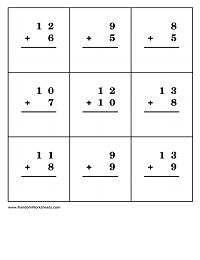This worksheet presents two numbers and an arithmetic operator along with a blank space in which to write the correct answer.

Lower Limit:
Upper Limit:
Total Pages:

Instructions

Lower Limit: the lower limit of the learning objective (i.e. the lowest number to show). This is typically set to 1, 5, 10, or 15. This number must be less than the Upper Limit.

Upper Limit: the upper limit of the learning objective (i.e. the highest number to show). This is typically set to 10, 15, or 20. This number must be greater than the Lower Limit.

Addition / Subtraction / Multiplication: Types of arithmetic problems to show (i.e. if Addition is the only box checked, then only Addition problems will be shown).

Total Pages: the total number of pages to generate. If printing double-sided (duplex), set this to an even number so that the last page prints on both sides.

Generate PDF: use this button to generate a printable PDF file of the worksheet pages with the desired learning objectives.

Show as Web Page: use this button if you do not have a PDF viewer and would like to display the worksheet pages as a single web page. You will need to manually adjust page margins when printing using this option.

Common Core Addition Worksheet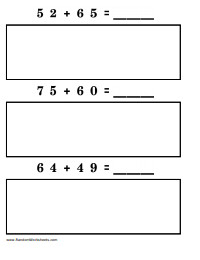This worksheet presents two numbers and an addition operator along with a large empty box in which to show work by counting "tens" and "ones" per Common Core methodology.

Lower Limit:
Upper Limit:
Total Pages:

Instructions

Lower Limit: the lower limit of the learning objective (i.e. the lowest number to show). This number must be less than the Upper Limit.

Upper Limit: the upper limit of the learning objective (i.e. the highest number to show). This number must be greater than the Lower Limit.

Total Pages: the total number of pages to generate. If printing double-sided (duplex), set this to an even number so that the last page prints on both sides.

Generate PDF: use this button to generate a printable PDF file of the worksheet pages with the desired learning objectives.

Show as Web Page: use this button if you do not have a PDF viewer and would like to display the worksheet pages as a single web page. You will need to manually adjust page margins when printing using this option.

Common Core Comparison Worksheet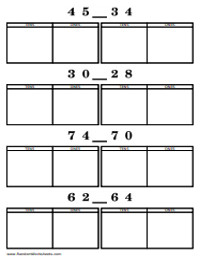This worksheet presents two numbers and a blank space in which to enter the comparison operator (<, >, =), along with two large empty boxs in which to show work by counting "tens" and "ones" for each number per Common Core methodology.

Lower Limit:
Upper Limit:
Total Pages:

Instructions

Lower Limit: the lower limit of the learning objective (i.e. the lowest number to show). This number must be less than the Upper Limit.

Upper Limit: the upper limit of the learning objective (i.e. the highest number to show). This number must be greater than the Lower Limit.

Total Pages: the total number of pages to generate. If printing double-sided (duplex), set this to an even number so that the last page prints on both sides.

Generate PDF: use this button to generate a printable PDF file of the worksheet pages with the desired learning objectives.

Show as Web Page: use this button if you do not have a PDF viewer and would like to display the worksheet pages as a single web page. You will need to manually adjust page margins when printing using this option.

Common Core Comparison Worksheet (Alternative Format 1)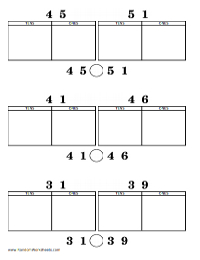This worksheet presents two numbers along with two large empty boxes in which to show work by counting "tens" and "ones" for each number per Common Core methodology. The numbers are then repeated with a circle between them in which to enter the comparison operator (<, >, =).

Lower Limit:
Upper Limit:
Total Pages:

Instructions

Lower Limit: the lower limit of the learning objective (i.e. the lowest number to show). This number must be less than the Upper Limit.

Upper Limit: the upper limit of the learning objective (i.e. the highest number to show). This number must be greater than the Lower Limit.

Total Pages: the total number of pages to generate. If printing double-sided (duplex), set this to an even number so that the last page prints on both sides.

Generate PDF: use this button to generate a printable PDF file of the worksheet pages with the desired learning objectives.

Show as Web Page: use this button if you do not have a PDF viewer and would like to display the worksheet pages as a single web page. You will need to manually adjust page margins when printing using this option.

Common Core Comparison Worksheet (Alternative Format 2)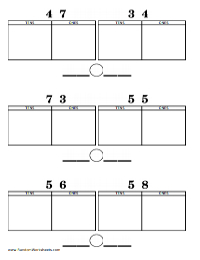This worksheet presents two numbers along with two large empty boxes in which to show work by counting "tens" and "ones" for each number per Common Core methodology. Two blanks are then presented with a circle between them in which to enter the original numbers and the comparison operator (<, >, =).

Lower Limit:
Upper Limit:
Total Pages:

Instructions

Lower Limit: the lower limit of the learning objective (i.e. the lowest number to show). This number must be less than the Upper Limit.

Upper Limit: the upper limit of the learning objective (i.e. the highest number to show). This number must be greater than the Lower Limit.

Total Pages: the total number of pages to generate. If printing double-sided (duplex), set this to an even number so that the last page prints on both sides.

Generate PDF: use this button to generate a printable PDF file of the worksheet pages with the desired learning objectives.

Show as Web Page: use this button if you do not have a PDF viewer and would like to display the worksheet pages as a single web page. You will need to manually adjust page margins when printing using this option.

Subscribe to our Newsletter

By clicking "Subscribe" I agree that:

Acknowledgements

Openclipart for Open Source clipart.
TCPDF FLOSS PHP class for generating PDF documents.
Apache OpenOffice The Free and Open Productivity Suite.
OpenNewsletter 2.5 Open Source newsletter and mailing-list manager.